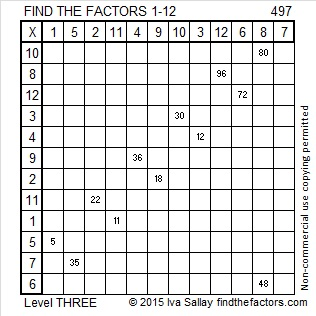# 497 and Level 3

497 is the sum of a five consecutive prime numbers: 89 + 97 + 101 + 103 + 107 = 497.Print the puzzles or type the solution on this excel file: 12 Factors 2015-05-18

—————————————————————————————————

• 497 is a composite number.
• Prime factorization: 497 = 7 x 71
• The exponents in the prime factorization are 1 and 1. Adding one to each and multiplying we get (1 + 1)(1 + 1) = 2 x 2 = 4. Therefore 497 has exactly 4 factors.
• Factors of 497: 1, 7, 71, 497
• Factor pairs: 497 = 1 x 497 or 7 x 71
• 497 has no square factors that allow its square root to be simplified. √497 ≈ 22.2934968—————————————————————————————————

A Logical Approach to solve a FIND THE FACTORS puzzle: Find the column or row with two clues and find their common factor. Write the corresponding factors in the factor column (1st column) and factor row (top row).  Because this is a level three puzzle, you have now written a factor at the top of the factor column. Continue to work from the top of the factor column to the bottom, finding factors and filling in the factor column and the factor row one cell at a time as you go.This site uses Akismet to reduce spam. Learn how your comment data is processed.